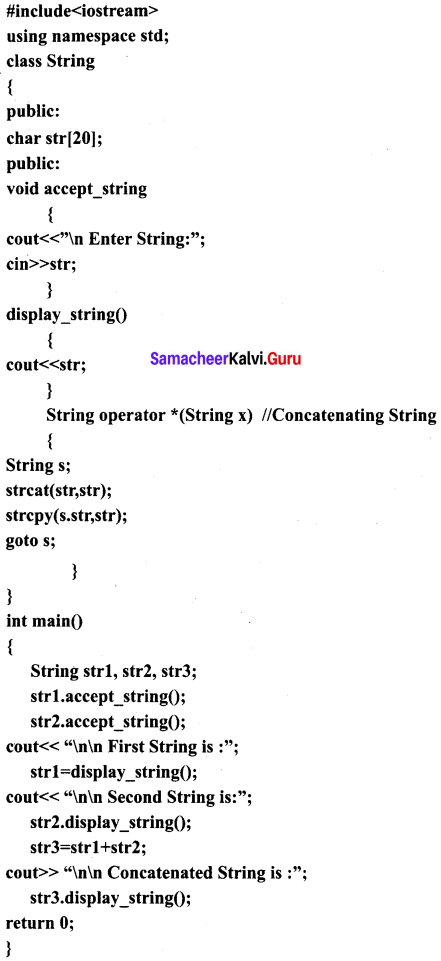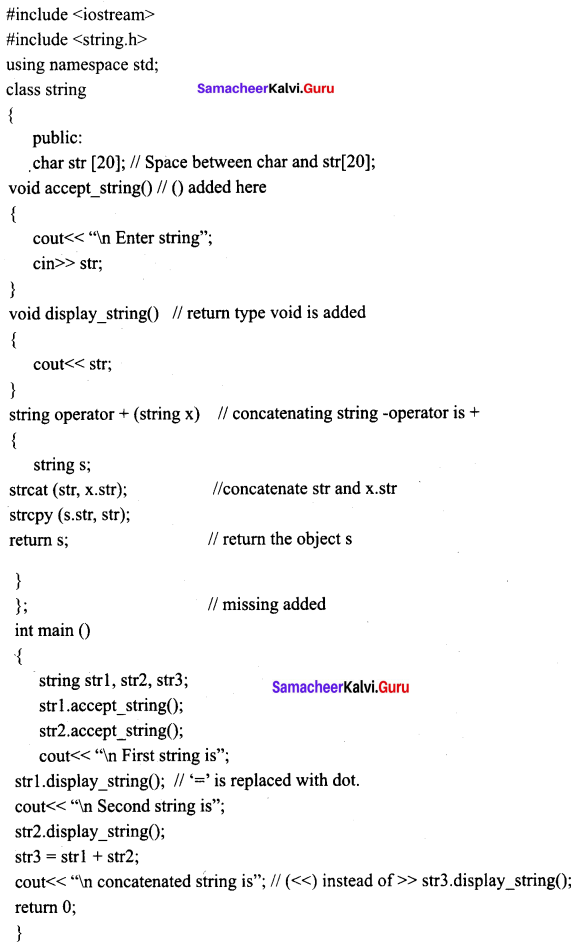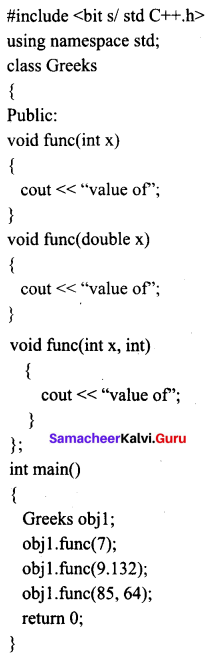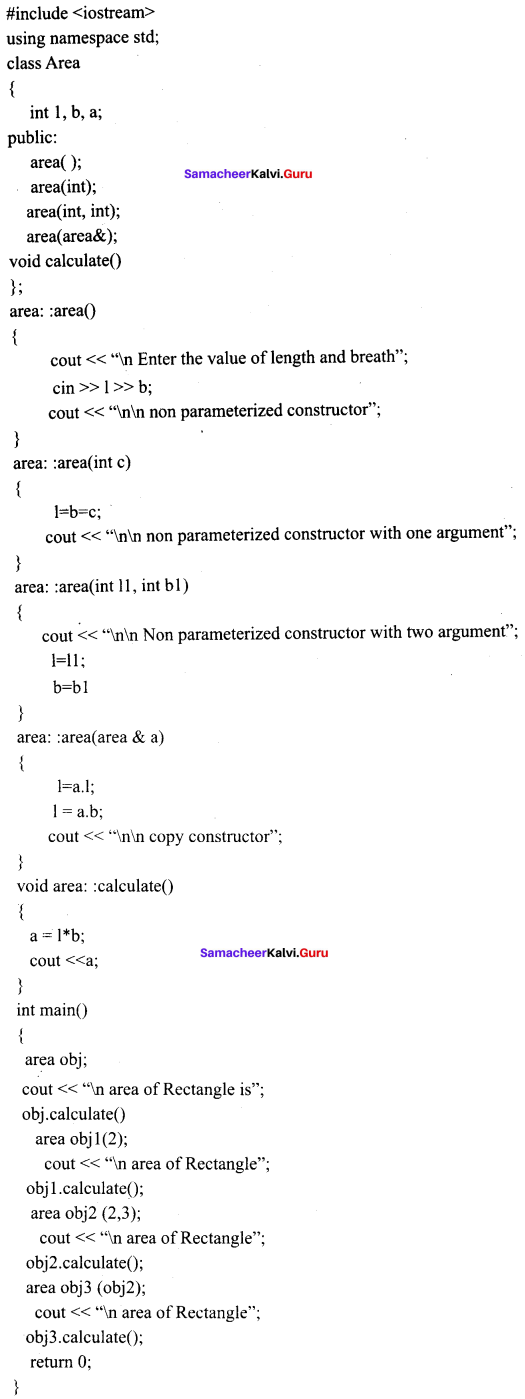# Samacheer Kalvi Books: Tamilnadu State Board Text Books Solutions

## Samacheer Kalvi 11th Computer Science Chapter 15 Polymorphism Notes PDF Download: Tamil Nadu STD 11th Computer Science Chapter 15 Polymorphism NotesSamacheer Kalvi 11th Computer Science Chapter 15 Polymorphism Notes PDF Download: Tamil Nadu STD 11th Computer Science Chapter 15 Polymorphism Notes

## Samacheer Kalvi 11th Computer Science Chapter 15 Polymorphism Notes PDF Download

We bring to you specially curated Samacheer Kalvi 11th Computer Science Chapter 15 Polymorphism Notes PDF which have been prepared by our subject experts after carefully following the trend of the exam in the last few years. The notes will not only serve for revision purposes, but also will have several cuts and easy methods to go about a difficult problem.

 Board Tamilnadu Board Study Material Notes Class Samacheer Kalvi 11th Computer Science Subject 11th Computer Science Chapter Chapter 15 Polymorphism Format PDF Provider Samacheer Kalvi Books

## How to Download Samacheer Kalvi 11th Computer Science Chapter 15 Polymorphism Notes PDFs?

2. Click on the Samacheer Kalvi 11th Computer Science Notes PDF.
3. Look for your preferred subject.
4. Now download the Samacheer Kalvi 11th Computer Science Chapter 15 Polymorphism notes PDF.

## Download Samacheer Kalvi 11th Computer Science Chapter 15 Polymorphism Chapterwise Notes PDF

PART – 1

Question 1.
Which of the following refers to a function having more than one distinct meaning?

Question 2.
Which of the following reduces the number of comparisons in a program?

Question 3.
void dispchar(char ch = ‘\$\ int size=10)
{

for(int i = 1;i< = size;i ++)
cout << ch;

}
How will you invoke the function dispchar() for the following input?
To print \$ for 10 times
(a) dispchar();
(b) dispchar(ch,size);
(c) dispchar(\$,10);
(d) dispchar(T, 10 times);
(c) dispchar(\$,10);

Question 4.
Which of the following is not true with respect to function overloading?
(a) The overloaded functions must differ in their signature.
(d) Destructor function cannot be overloaded.
(d) Destructor function cannot be overloaded.

Question 5.
(a) void fun (intx);
void fun (char ch);
(b) void fun (intx);
void fun (inty);
(c) void fun (double d);
void fun (char ch);
(d) void fun (double d);
void fun (inty);
(a) void fun (intx);
void fun (char ch);

Question 6.
Which of the following function(s) combination cannot be considered as overloaded function(s) in the given snippet?
void print(char A,int B); // F1
void printprint(int A, float B); // F2
void Print(int P=10); // F3
void printQ; // F4
(a) F1, F2, F3, F4
(b) F1, F2, F3
(c) F1, F2, F4
(d) F1, F3, F4
(d) F1, F3, F4

Question 7.
Which of the following operator is by default overloaded by the compiler?
(a) *
(b) +
(c) + =
(d) = =
Based on the following program answer the questions (8) to (10)
#include
using namespace std;
class Point {
private:
int x, y; .
public:
Point(int x1,int y1)
{

x=x1;y=y1;

}
void operator+(Point & pt3);
void show() {cout << “x =” << x << “y =” << y; }
};
void Point: :operator + (Point & pt3)
{

x+=pt3.x;
y += pt3.y;

}
int main()
{

Point pt1(3, 2),pt2(5, 4);
pt1+pt2;
pt1.show();
return 0;
}

(b) +

Question 8.
Which of the following operator is overloaded?
(a) +
(b) Operator
(c) : :
(d) =
(a) +

Question 9.
(a) pt1 + pt2;
(b) Point pt1(3, 2),pt2(5, 4);
(c) ptl.show();
(d) return 0;
(d) return 0;

Question 10.
What is the output for the above program?
(a) x = 8, y = 6
(b) x = 14, y = 14
(c) x = 8, y = 6
(d) x = 5, y = 9
(d) x = 5, y = 9

PART – 2
II. Answers to all the questions

Question 1.
The ability of the function to process the message or data in more than one form is called as function overloading. In other words function overloading means two or more functions in the same scope share the same name but their parameters are different.

Question 2.
List the operators that cannot be overloaded.
Operator that are not overloaded are follows:

1. scope operator ::
2. sizeof
3. member selector.
4. member pointer selector *
5. ternary operator ?:

Question 3.
class add{int x; public: add(int)}; Write an outline definition for the constructor.
{

x = temp;

}

Question 4.
Does the return type of a function help in overloading a function?

Question 5.
Function overloading is not only implementing polymorphism but also reduces the number of comparisons in a program and makes the program to execute faster. It also helps the programmer by reducing the number of function names to be remembered.

PART – 3
III. Answers to all the questions

Question 1.

1. The overloaded function must differ in the number of its arguments or data types.
3. The default arguments of overloaded functions are not considered as part of the parameter list in function overloading.

Question 2.
How does a compiler decide as to which function should be invoked when there are many functions? Give an example.
When you call an overloaded function (when there are many functions with same name), the compiler determines the most appropriate definition to use by comparing the argument types used to call the function with the parameter types specified in the definitions. The process of selecting the most appropriate overloaded function or operator is called overload resolution.
Example:
#include using namespace std; void print (int i)
{

cout<< “It is integer” <<i<< endl;

}

void print (string c)

{

cout<< “It is string”<< c << endl;

}
int main ()
{

print (10);
print (“Good”);
return 0;

}
Output:
It is integer 10
It is string Good

Question 3.
The term operator overloading, refers to giving additional functionality to the normal C++ operators like It is also a type of polymorphism in which an operator is overloaded to give user defined meaning to it.

For example ‘+’ operator can be overloaded to perform addition on various data types, like for Integer, String (concatenation) etc.

Question 4.
Function overloading can be applied for constructors, as constructors are special functions of classes. A class can have more than one constructor with different signature. Constructor overloading provides flexibility of creating multiple type of objects for a class.

1. Memory is allocated for the objects.
2. Initialisation for the objects.

Question 5.
class sale ( int cost, discount ;public: sale(sale &); Write a non inline definition for constructor specified;
class sale
{

int cost, discount;
public:
sale (sale&);

};
// non inline constructor
sale: : sale(sale&s)
{

cost = s.cost;
discount = s.discount;

}

PART – 4
IV. Answers to all the questions

Question 1.

1. Precedence and Associativity of an operator cannot be changed.
2. No new operators can be created, only existing operators can be overloaded.
3. Cannot redefine the meaning of an operator’s procedure. You cannot change how integers are added. Only additional functions can be to an operator.
4. Overloaded operators cannot have default arguments.
5. When binary operators are overloaded, the left hand object must be an object of the relevant class.

Question 2.
Answer the question (i) to (v) after going through the following class.
classBook
{
intBookCode ; char Bookname;float fees;
public:
Book() //Function 1
{
fees = 1000;
BookCode = 1;
strcpy (Bookname, “C++”);
}
void display(float C) //Function 2
{
cout << BookCode << “:”<< Bookname << “:”<< fees << endl;
~ Book() //Function 3
{
cout << “End of Book Object”<< endl;
}

Book (intSC, char S[ ], float F); //Function 4

};
1. In the above program, what are Function 1 and Function 4 combined together referred as?
2. Which concept is illustrated by Function3? When is this function called/ invoked?
3. What is the use of Function3?
4. Write the statements in main to invoke functionl and function2
5. Write the definition for Function4.

1. Constructor overloading (function 1 and function 4 are constructors with different signatures in the class book)
2. Function 3 is destructor of the class. Function 3 is executed when the object of the class book goes out of scope.
3. Function 3 is destructor of the class.
• Destructor (function3) will free resources if any that the object may have acquired during its lifetime
• Destructor function removes the memory of an object which was allocated by the constructor at the time of creating an object. Thus frees the unused memory.
4. book b;
b.display (4.5);
5. Book: :Book (int sc.char.s[], float, F) // Function 4
{
Book Code = SC
strcpy (Book name, S);
fees = F;
}

Question 3.
Write the output of the following program.
include
using namespace std;
class Seminar
{
int Time;
public:
Seminar()
{

Time = 30; cout << “Seminar starts now” << endl;

}
void Lecture()
{
cout << “Lectures in the seminar on”<< endl;
}
Seminar(int Duration)
{

Time=Duration;cout<< “Welcome to Seminar”<<endl;

}
Seminar(Seminar &D)
{

Time=D.Time;cout<< “Recap of Previous Seminar Content”<<endl;

}
~Seminar()
{
cout<< “Vote of thanks”<<endl;
}
};
int main()
{

Seminar s1, s2(2), s3(s2);
s1.Lecture();
return 0;

}
Output:
Seminar starts now
Welcome to seminar
Recap of previous seminar content
Lectures in the seminar on
Vote of thanks
Vote of thanks
Vote of thanks

Question 4.
Debug the following program.Corrected ProgramOutput
Enter string: God
Enter string: Bless
First string is: God
Second string is: Bless
Concatenated string is: God Bless

Question 5.
Answer the questions based on the following program
#include
#include
using namespace std;
class comp
{
public:
char;
void getstring(char str)
{
strcpy(s,str);
}
void operator = = (comp);
};
void comp::operator = = (comp ob)
{
if(strcmp(s,ob.s) = = 0)
cout << “\nStrings are Equal”;
else
cout<< “\nStrings are not Equal”;
}
int main()
{
comp ob, ob1;
char stringl, string2;
cout << “Enter First String:”; cin >> string1;
ob.getstring(string1);
cout<< “\n Enter Second String:”; cin >> string2;
ob1.getstring(string2);
ob = = obi;
return 0;
}
(i) Mention the objects which will have the scope till the end of the program.
(ii) Name the object which gets destroyed in between the program.
(iii) Name the operator which is over loaded and write the statement that invokes it.
(iv) Write out the prototype of the overloaded member function.
(v) What types of operands are used for the overloaded operator?
(vi) Which constructor will get executed? Write the output of the program.
(i) Objects: ob and obi of main ()
(ii) object ob of void operator = = (comp ob);
Statement that invokes: ob = = ob1;
(v) void operator = = (comp ob):
(v) Operands used are the objects of the class comp
(vi) The default constructor generated by the compiler will be executed.[comp();]

Output 1
Enter first string: hello Enter second string: hello String are equal

Output 2
Enter first string: hello Enter second string: fine String are not equal.

PART – 1

Question 1.
to be displayed in more than one form.
a) Polymorphism
b) Inheritance
c) Encapsulation
d) Data abstraction
a) Polymorphism

Question 2.
The process of selecting the most appropriate overloaded function or operator is called …………………
(b) prototype
(c) polymorphism

Question 3.
_________ The ability of the function to process the message or data in more than one form is called overloading.
a) Function
b) Operator
c) Operand
d) Either A or B
a) Function

Question 4.
………………… cannot have default arguments.
(d) prototype

Question 5.
The process of selecting the most appropriate overloaded function or operator is called _________
d) None of these

Question 6.
(a) *
(b) + = =
(c) +
(d) C++
(d) C++

Question 7.
______ overloading helps the programmer by reducing the number of function names to be remembered.
a) Function
b) Operator
c) Operand
d) Either A or B
a) Function

PART – 2

Question 1.
The ability of the function to process the message or data in more than one form is called function overloading.

Question 2.
#include
using namespace std;
void print(int i)
{cout << “It is integer” << i <<endl;}
void print(double f)
{ cout << “It is float” << f <<endl;}
void print(string c)
{ cout << “It is string” << c <<endl;}
int main() {

print(10);
print(10.10);
print(“Ten”);
return 0;

}
Output:
It is integer 10
It is float 10.1
It is string Ten

Question 3.
The term overloading means a name having two or more distinct meanings. Thus an ‘overloaded function’ refers to a function having more than one distinct meaning.

PART – 3

Question 1.
What is function signature?
The ability of the function to process the message or data in more than one form is called as function overloading. It implies that two or more functions in the same scope share the same name but their parameters are different. In this situation, the functions that share the same name are said to be overloaded and the process is called function overloading. The number and types of a function’s parameters are called the function’s signature.

Question 2.
Function overloading can be applied for constructors, as constructors are special functions of classes. A class can have more than one constructor with a different signature. Constructor overloading provides the flexibility in creating multiple types of objects for a class.

PART – 4
IV. Explain in Detail

Question 1.
Give the output of the following program.Output:
value of x is 7
value of x is 9.132
value of x and y is 85,64

Question 2.
WrIte a program to Implement constructor ovedodtng.
Program:
#include<iostream>
using namespace std;
{
int num1, num2, sum;
public:
{
cout<<“\n Constructor without
parameters..”;
num1= 0;
num2= 0;
sum = 0;
}
add (int s1, int s2 )
{
C0ut<<“\n Parameterized
constructor…”;
num1= s1;
num2=s2;
sum=0;
}
{
cout<<“\n Copy Constructor…”;
num1= a.num1;
num2=a.num2;
sum = 0;
}
void getdata()
{
cout<<“\nEnter data … “;
cin>>num1>>num2;
}
{
sum=num1+num2;
}
void putdata()
{
cout<<“\n The numbers are..”;
cout<<numl<<‘\t'<<num2;
cout<<“\n The sum of the numbers are.. “<< sum;
}
};
int main()
{
add a, b (10, 20), c(b);
a. getdata();
cout<<“\n Object a : “;
a. putdata();
cout<<“\n Object b : “;
b. putdata();
cout<<“\n Object c..”;
c. putdata();
return 0; .
}

Output :
Constructor without parameters..
Parameterized constructor…
Copy Constructor …
Enter data … 20 30
Object a :
The numbers are..20 30
The sum of the numbers are.. 50
Object b :
The numbers are..10 20
The sum of the numbers are.. 30
Object c..
The numbers are..10 20
The sum of the numbers are.. 30

Question 3.
Write a program to find the area of a rectangle using constructor overloading in a class.Output:
Enter the value of length and breadth 10 20
Non parameterized constructor
area of Rectangle is 60
Parameterized constructor with one argument
area of Rectangle 8
Parameterized constructor with two argument
area of Rectangle 10
copy constructor
area of Rectangle 10

## How to Prepare using Samacheer Kalvi 11th Computer Science Chapter 15 Polymorphism Notes PDF?

Students must prepare for the upcoming exams from Samacheer Kalvi 11th Computer Science Chapter 15 Polymorphism Notes PDF by following certain essential steps which are provided below.

• Use Samacheer Kalvi 11th Computer Science Chapter 15 Polymorphism notes by paying attention to facts and ideas.
• Pay attention to the important topics
• Refer TN Board books as well as the books recommended.
• Correctly follow the notes to reduce the number of questions being answered in the exam incorrectly
• Highlight and explain the concepts in details.

## Frequently Asked Questions on Samacheer Kalvi 11th Computer Science Chapter 15 Polymorphism Notes

#### How to use Samacheer Kalvi 11th Computer Science Chapter 15 Polymorphism Notes for preparation??

Read TN Board thoroughly, make separate notes for points you forget, formulae, reactions, diagrams. Highlight important points in the book itself and make use of the space provided in the margin to jot down other important points on the same topic from different sources.

#### How to make notes for Samacheer Kalvi 11th Computer Science Chapter 15 Polymorphism exam?

Read from hand-made notes prepared after understanding concepts, refrain from replicating from the textbook. Use highlighters for important points. Revise from these notes regularly and formulate your own tricks, shortcuts and mnemonics, mappings etc.
Share: